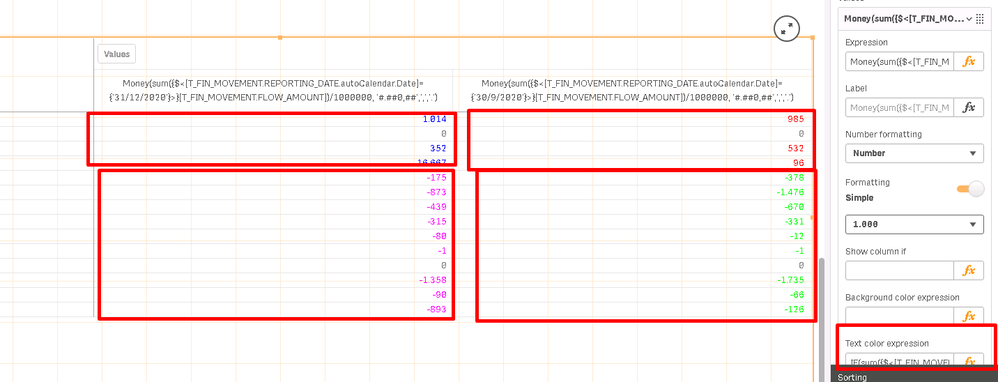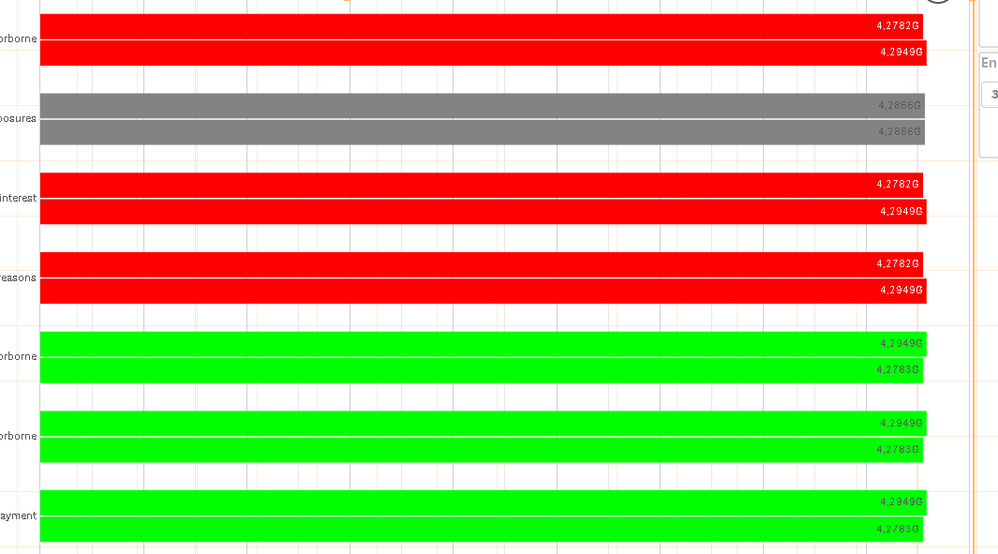Announcements
Applications are open for the 2024 Qlik Luminary Program. Apply by December 15 here.
cancel
Showing results for
Did you mean:Contributor III

## Color code by expression the bars of the bar-plot using 4 color-codes

I have created the barplot below in Qlik Sense Service:bar plot with 2 color codes instead of 4

This barplot has two measures. The expression per measure is:

Measure 1: sum({\$<[REPORTING_DATE.autoCalendar.Date]={'28/08/1999'}>}[FLOW_AMOUNT])

Measure 2: sum({\$<[REPORTING_DATE.autoCalendar.Date]={'28/10/1999'}>}[FLOW_AMOUNT])

So, the left (upper) bar computes the amount sum per Customer for 28/08/1999  while the second right bar computes for the same customer the sum amount for 28/10/1999.

I would like to color code by expression each bar using 4 different conditional color expressions.

To do so I wrote the color code expression below in the appearance tab of the bar chart:

IF(sum({\$<[REPORTING_DATE.autoCalendar.Date]={'28/10/1999'},[FLOW_TYPE]={'INFLOW'}>}[FLOW_AMOUNT]), LightRed(),

IF(sum({\$<[REPORTING_DATE.autoCalendar.Date]={'28/08/1999'}, [FLOW_TYPE]={'INFLOW'}>}[FLOW_AMOUNT]), LightBlue(),

IF(sum({\$<[REPORTING_DATE.autoCalendar.Date]={'28/10/1999'}, [FLOW_TYPE]={'OUTFLOW'}>}[FLOW_AMOUNT]), LightGreen(),

IF(sum({\$<[REPORTING_DATE.autoCalendar.Date]={'28/08/1999'}, [FLOW_TYPE]={'OUTFLOW'}>}[FLOW_AMOUNT]), LightMagenta(),
DarkGray()))))

Based on the screenshot above the LightBlue() columns and LightMagenta() are never colored, but rather they inherit the color of LightGreen() and LightRed().

I simulated the same experiment in a pivot table and I successfully managed to color the text as expected. See below:Pivot table with 4 color codes

In the pivot I managed to color each sum amount per customer based on the two measures and the 4 color conditions I wanted.

The text color expression is as follows:

Measure 1 text color expression-only relevant to date 28/08/1999:

IF(sum({\$<[REPORTING_DATE.autoCalendar.Date]={'28/08/1999'}, [FLOW_TYPE]={'INFLOW'}>}[FLOW_AMOUNT]), LightBlue(),

IF(sum({\$<[REPORTING_DATE.autoCalendar.Date]={'28/08/1999'}, [FLOW_TYPE]={'OUTFLOW'}>}[FLOW_AMOUNT]), LightMagenta(), DarkGray()))

Measure 2 text color expression-only relevant to date 28/10/1999:

IF(sum({\$<[REPORTING_DATE.autoCalendar.Date]={'28/10/1999'}, [FLOW_TYPE]={'INFLOW'}>}[FLOW_AMOUNT]), LightRed(),

IF(sum({\$<[REPORTING_DATE.autoCalendar.Date]={'28/10/1999'}, [FLOW_TYPE]={'OUTFLOW'}>}[FLOW_AMOUNT]), LightGreen(), DarkGray()))

So my question is how can I make the bar plot bars to have the same behavior as the pivot table bars. Unfortunately, as I have figured out so far the Bar plot does not allow to color measures individually by expression, but the pivot table on the contrary allowed to me to color measures individually by expression.

Labels (1)
• ### Chart

1 Solution

Accepted SolutionsContributor III
Author

Satish I have solved my problem using 2 dimensions in the bar chart, with the second dimension to be a value list. So I ended up to create 4 if statements based on the 2 dimensions. Each if statement had a different color. That approach worked as expected. Kudos to this question.

3 RepliesContributor II

Hello Pal,

As per question  bar chart picture, i can understand that  you have two measure in chart.

1st measure is having +ve value for inflow or outflow and vice versa for -ve value.

same goes for 2nd measure.

what i can understand is that color code 1 bar and color code 3 bar showing value from  measure 1

and similarly color code 2 bar and color code 4 bar showing value from measure 2.

If above assumption is correct,

you can below two expression in you two of measure to set color code 1 for +ve value and color code 2 for -ve value.

measure 1:

IF(sum({\$<[REPORTING_DATE.autoCalendar.Date]={'28/08/1999'}, [FLOW_TYPE]={'INFLOW'}>}[FLOW_AMOUNT])>0, LightBlue(),

IF(sum({\$<[REPORTING_DATE.autoCalendar.Date]={'28/08/1999'}, [FLOW_TYPE]={'OUTFLOW'}>}[FLOW_AMOUNT])<0, LightMagenta(), DarkGray()))

measure 2:

IF(sum({\$<[REPORTING_DATE.autoCalendar.Date]={'28/10/1999'}, [FLOW_TYPE]={'INFLOW'}>}[FLOW_AMOUNT])>0, LightGreen(),

IF(sum({\$<[REPORTING_DATE.autoCalendar.Date]={'28/10/1999'}, [FLOW_TYPE]={'OUTFLOW'}>}[FLOW_AMOUNT])<0, LightRed(), DarkGray()))

Please let me know if face any issue.

Hope this will work for you smoothly.

ThanksContributor III
Author

Hello @satish3922  and ty for the reply. I have tested your proposal and it didn't work out for me.

Basically, in the barplot if I replace the measure (which represents the height of the bar) with each of the expressions below:

1) Measure 1 (bar 1):

IF(sum({\$<[REPORTING_DATE.autoCalendar.Date]={'28/08/1999'}, [FLOW_TYPE]={'INFLOW'}>}[FLOW_AMOUNT])>0, LightBlue(),

IF(sum({\$<[REPORTING_DATE.autoCalendar.Date]={'28/08/1999'}, [FLOW_TYPE]={'OUTFLOW'}>}[FLOW_AMOUNT])<0, LightMagenta(), DarkGray()))

and 2) Measure 2 (bar 2):

IF(sum({\$<[REPORTING_DATE.autoCalendar.Date]={'28/10/1999'}, [FLOW_TYPE]={'INFLOW'}>}[FLOW_AMOUNT])>0, LightGreen(),

IF(sum({\$<[REPORTING_DATE.autoCalendar.Date]={'28/10/1999'}, [FLOW_TYPE]={'OUTFLOW'}>}[FLOW_AMOUNT])<0, LightRed(), DarkGray()))

I get in return the bar chart below:Bar plot after replacing each measure

In the above bar chart I have lost the summary amount per customer and all customers have the same amount, which is not correct. Could you please help me on this?Contributor III
Author

Satish I have solved my problem using 2 dimensions in the bar chart, with the second dimension to be a value list. So I ended up to create 4 if statements based on the 2 dimensions. Each if statement had a different color. That approach worked as expected. Kudos to this question.Tags
Community Browser